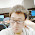### leetcode Question 69: Permutations

Permutations

Given a collection of numbers, return all possible permutations.
For example,
[1,2,3] have the following permutations:
[1,2,3][1,3,2][2,1,3][2,3,1][3,1,2], and [3,2,1].

Analysis:
The idea of this classic problem is to use backtracking.
We want to get permutations, which is mainly about swap values in the list.
Consider:
a --> a
ab --> ab, ba
abc --> abc, acb, bac, bca, cab, cba.
...
where for the length of n, the permutations can be generated by
(1) Swap the 1st element with all the elements, including itself.
(2) Then the 1st element is fixed, go to the next element.
(3) Until the last element is fixed. Output.
It's more clear in the figure above. The key point is to make the big problem into smaller problem, here is how to convert the length n permutation into length n-1 permutation problem.

Code:
class Solution {
public:

void perm(vector<int> num,int k,int n, vector<vector<int> > &res){
if (k==n){
res.push_back(num);
}else{
for (int i=k;i<=n;i++){
int tmp = num[k];
num[k]=num[i];
num[i]=tmp;

perm(num,k+1,n,res);

tmp = num[k];
num[k]=num[i];
num[i]=tmp;
}
}
}

vector<vector<int> > permute(vector<int> &num) {
// Start typing your C/C++ solution below
// DO NOT write int main() function
vector<vector<int> > res;
perm(num,0,(num.size()-1),res);
return res;
}
};


1.Hi,

I think the second swap in perm function is not necessary. You just modified a copy of num variable, you don't need to get them back.

1.I think the second swap is the stantard structure of backtracking algorithm, where you change the status, after recursion, you change the status back. A better algorithm is the N-queen problem, you can have a better understanding.

For this problem, I think you can see the figure. The 2nd level of tree structure, if you do not swap back, the next node in the 2nd level cannot get the correct form. Hope this explanation can help you. Thanks!

2.This comment has been removed by the author.

2.how do you deal with when duplicates exist?

1.Generally speaking, when you meet one element which has occurred in previous, just skip it and do the next element.

Details please check this post :
http://yucoding.blogspot.com/2013/04/leetcode-question-70-permutations-ii.html

3.Thank you for your neat solution. I have tried submitting your answer without line15~line17 to OJ, and it has been accepted. I guess the second swap will also generate the right answer, but not necessary.

4.This comment has been removed by the author.

5.Hi, Yu,

Nice and neat solution.

I have a quick comment. Since you swap them back with the second swap. You could simply pass num by reference instead of by value.

Best,
Tony

6.The second swap can never be executed, so it is useless?